# Using Trig Ratios to Solve Triangles: Sides

The trig ratios can be used to find lots of information, and one of their main purposes is to help solve triangles. To solve a triangle means to find the length of all the sides and the measure of all the angles.

This lesson will cover how to use trig ratios to find the side lengths of a triangle.

There are three steps:

1. Choose which trig ratio to use.
- Choose either sin, cos, or tan by determining which side you know and which side you are looking for.

2. Substitute
- Substitute your information into the trig ratio.

3. Solve
- Solve the resulting equation to find the length of the side.

Example: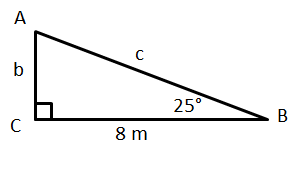1. Find b.

Step 1: Choose which trig ratio to use.

First, we know we must look at angle B because that is the angle we know the
measure of.(Now, you could find the measure of angle A and then use that but
that's less reliable since you could make a mistake.)

So, looking at angle B, we want to identify which sides are involved. We know
one side is 8m, and that side is adjacent to angle B. The side we're looking
for is opposite angle B. So we need to choose the trig ratio that has
opposite and adjacent. This of course is the tangent.

Step 2: Substitute

Next, we write our trig ratio:Then, we substitute in the angle
and the side we know: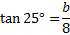Step 3: Solve

Now move the 8 to the other side
by multiplying both sides by 8: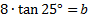And use a calculator to find the answer.
Well round to the nearest tenth: 3.7 m.

2. Find c.

Now that we know two sides, you could use the Pythagorean Theorem
to find the third. But that's less reliable because
if you made a mistake on side b, then side c will also be wrong.
So we are going to repeat the same process for side c.

Step 1: Choose the trig ratio to use.

We're still using angle B. 8m is the adjacent and c is the hypotenuse.
The trig ratio that uses the adjacent and hypotenuse is the cosine.

Step 2: Substitute

Write our trig ratio:Then, we substitute in the
angle and the side we know:Step 3: Solve

Since our variable is on the
bottom, we can start by
cross multiplying: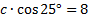Then we'll divide
both sides by cos 25°: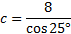And use a calculator to
find the answer. Well round
to the nearest tenth:    8.8 m.

Note: If your calculator doesn't seem to be giving you the right answer, read your manual or ask someone for help. Each calculator is different, and you have to enter the information in the correct order to get the right answer. Also, make sure your calculator is in Degree mode (not Radians)

Practice:

Find the following. Round your answers to the nearest tenth.

1) a      2) c      3) x      4) y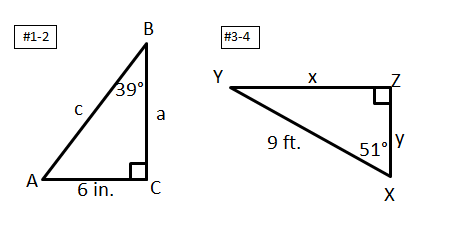Answers: 1) 7.4 in   2) 9.5 in.  3) 7.0 ft    4) 5.7 ft.

 Related Links: Math Fractions Factors